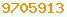# trigonometry : Java Glossary

trigonometry
Use the java.lang.Mathlang.Math class to compute trignometric functions. You say things like:
```// calc sine of angle theta, expressed in radians
double s = Math.sin( theta );```
You can leave off the Math. if you use a static import.
```static import java.lang.Math.*;
...
// calc sine of angle theta, expressed in radians
double s = sin( theta );```

For the most part, all the trig functions you need are in the java.lang.Math class with the same names mathematicians use namely: sin, cos, tan, asin, acos, atan, sinh, cosh, tanh.

• Warning! The trig functions work in radians not degrees. To convert radians ⇔ degrees, use Math. toDegrees and Math. toRadians.
• The sec, cot, csc, asec, acot and acsc functions are missing. You can create them with simple trignometric identities, e.g.:
• Use Math.E for e, the base of the natural logarithms and Math. PI for π.
• Java has two functions for computing arctangent: java.lang.Math.atan and atan2. They work in radians. The result of atan will always be range -pi/2 to +pi/2. The result of atan2 will be in the range -pi to +pi in the quadrant consistent with the signs of the two arguments.

## Learning More

Oracle’s Javadoc on java.lang.Math class : available:This page is postedon the web at: http://mindprod.com/jgloss/trigonometry.html Optional Replicator mirror of mindprod.com on local hard disk J: J:\mindprod\jgloss\trigonometry.htmlPlease read the feedback from other visitors, or send your own feedback about the site.Contact Roedy. Please feel free to link to this page without explicit permission. Canadian Mind Products IP:[65.110.21.43] Your face IP:[34.204.194.190] Feedback You are visitor number